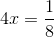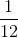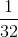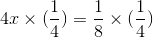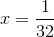# HSPT Math : How to solve one-step equations

## Example Questions

← Previous 1

### Example Question #1 : How To Solve One Step Equations

Solve for: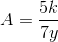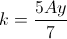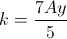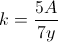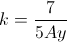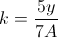Explanation: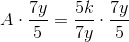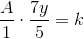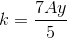### Example Question #2 : Algebra

In a math contest, the team from Lincoln High comprised five students - Melanie, Ned, Olivia, Phyllis, and Quinn. Melanie outscored Ned by 12 points; Ned outscored Quinn by 19 points; Phyllis outscored Ned by 45 points and Olivia by 20 points. Who came in third among the five?

Olivia

Phyllis

Quinn

Melanie

Ned

Melanie

Explanation:

Since this reasoning is valid regardless of the actual scores, suppose Quinn scored 100. Then Ned scored 19 more than Quinn, or 119, and Melanie scored 12 more than Ned, or 131. Phyllis scored 45 more than Ned, or 164, and Olivia scored 20 points fewer than Phyllis, or 144. This makes the order, from greatest to least:

Phyllis, Olivia, Melanie, Ned, Quinn

Melanie scored third among the five.

### Example Question #2 : How To Solve One Step Equations

Jasmine is participating in a swimming race. If the race is 3000 yards, what percentage of the race has she completed at the 2400 yardline?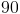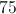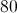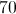Explanation:

Divide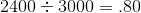.

Answer: Jasmine has completed 80% of the race.

### Example Question #2 : How To Solve One Step Equations

A full helium tank can blow up 50 balloons. At least how many times does the tank have to be re-filled in order to blow up 140 balloons?Explanation:

Divide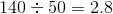Answer: The tank must be filled up at least 3 times.

### Example Question #7 : How To Solve One Step Equations

Solve: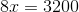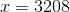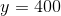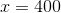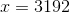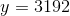Explanation:

To solve for, divide by eight on both sides of the equation.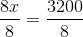### Example Question #2 : Algebra

Solve for: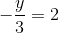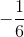Explanation:

To isolate the unknown variable, multiply both sides by negative three.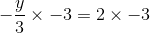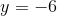### Example Question #9 : How To Solve One Step Equations

Solve: